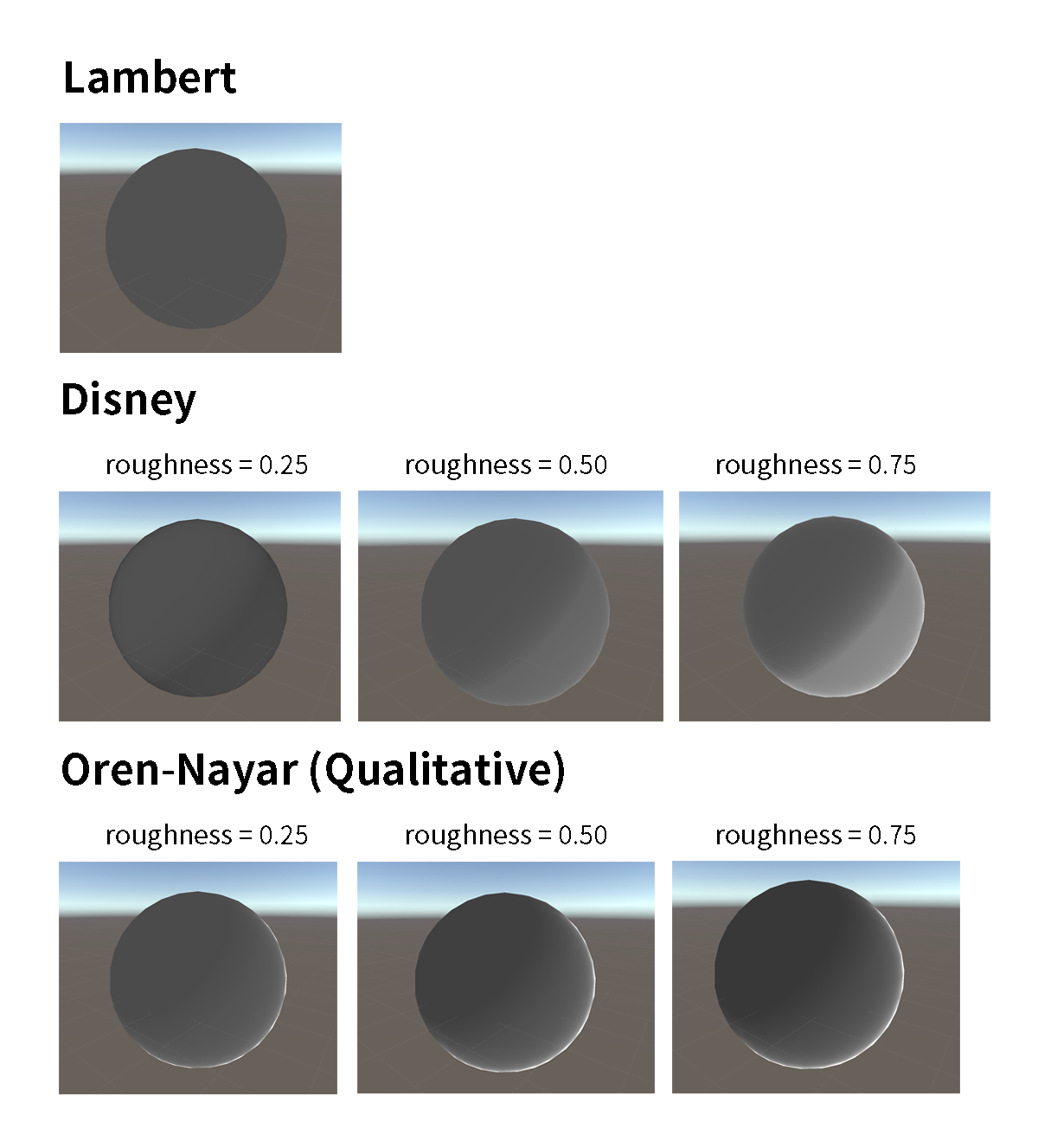## 光滑表面模型

$f_{\mathrm{diff}}(l, v) = \frac{\rho_{\mathrm{ss}}}{\pi}$

HLSL 实现：

$f_{\mathrm{diff}}(l, v) = (1 - F(l, h))\frac{\rho_{\mathrm{ss}}}{\pi}$

$f_{\mathrm{diff}}(l, v) = \frac{21}{20 \pi} \rho_{\mathrm{ss}} (1 - F_0) (1 - (1 - (n \cdot l)^+)^5)(1 - (1 - (n \cdot v)^+)^5)$

## 粗糙表面模型

### Disney Diffuse

Disney 原则的 BRDF 使用了如下的公式，Stevens 的教程中亦使用如下公式：

$f_{\mathrm{diff}} (l, v) = \frac{\rho_{\mathrm{ss}}}{\pi} (1 + (F_{D90} - 1)(1 - (n \cdot l)^+)^5) (1 + (F_{D90} - 1)(1 - (n \cdot v)^+)^5) \\ F_{D90} = 0.5 + 2 \sqrt{\alpha} (l \cdot h)^2$

HLSL 实现：

### Oren-Nayar

Oren-Nayar 模型基于微平面模型，选取了高斯分布的法线分布与 V 腔假设（V-cavity，轮廓是一片 V 型）的几何遮蔽阴影，每个微平面认为是符合 Lambert 公式。得到的结果如下：

$f_{\mathrm{diff}} (l, v) = \frac{\rho_{\mathrm{ss}}}{\pi} (A + B \frac{s}{t}) \\ A = 1 - 0.5\frac{\sigma^2}{\sigma^2 + 0.33} \\ B = 0.45\frac{\sigma^2}{\sigma^2 + 0.09} \\ s = (l \cdot v) - (n \cdot l) (n \cdot v) \\ t = \begin{cases} 1 & s \leq 0 \\ \max(n \cdot l, n \cdot v) & s > 0 \end{cases}$

$f_{\mathrm{diff}}(l, r) = \frac{\rho_{\mathrm{ss}}}{\pi} (A + B \max(0, \cos (\phi_i - \phi_r)) \sin \alpha \tan \beta) \\ \alpha = \max(\theta_i, \theta_r) \\ \beta = \min(\theta_i, \theta_r) \\$

$A = 1 - 0.5\frac{\sigma^2}{\sigma^2 + 0.33} + 0.17 \rho_{ss} \frac{\sigma^2}{\sigma^2 + 0.13}$

HLSL 实现：

### Hammon’s Diffuse BRDF

Oren-Nayar 的推导中使用的法线分布与几何遮蔽阴影模型并非是目前流行的 GGX NDF 与 Smith G，一种使用 GGX NDF 与 Smith 高度相关遮蔽阴影函数的推导结果是 Hammond 给出的如下公式：

$f_{\mathrm{diff}}(l, v) = \frac{\rho_{\mathrm{ss}}}{\pi} ((1 - \alpha) f_{\mathrm{smooth}} + \alpha f_{\mathrm{rough}} + \rho_{\mathrm{ss}} f_{\mathrm{multi}}) \\ f_{\mathrm{smooth}} = \frac{21}{20} (1 - F_0) (1 - (1 - (n \cdot l)^+)^5)(1 - (1 - (n \cdot v)^+)^5) \\ f_{\mathrm{rough}} = k_{\mathrm{facing}} (0.9 - 0.4 k_{\mathrm{facing}}) \frac{0.5 + (n \cdot h)}{n \cdot h} \\ k_{\mathrm{facing}} = 0.5 + 0.5 (l \cdot v) \\ f_{\mathrm{multi}} = 0.3641 \alpha$

## Unity 结果------ 本文结束，感谢您的阅读 ------